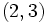# Endomorphism structure of symmetric group:S3

## Contents

View endomorphism structure of particular groups | View other specific information about symmetric group:S3

This article discusses the structure of the endomorphisms of symmetric group:S3, the symmetric group of degree three. We denote its elements as acting on the set$\{ 1,2,3 \}$, written using cycle decompositions, with composition by function composition where functions act on the left.

## Summary of information

Construct Value Order Second part of GAP ID (if group)
endomorphism monoid  ? 10 --
automorphism group symmetric group:S3 6 1
inner automorphism group symmetric group:S3 6 1
extended automorphism group dihedral group:D12 12 4
quasiautomorphism group dihedral group:D12 12 4
1-automorphism group dihedral group:D12 12 4
outer automorphism group trivial group 1 1

## Description of automorphism group

Symmetric group:S3 is a complete group (i.e., it is a centerless group and every automorphism is inner). See also symmetric groups on finite sets are complete.

Thus, all its automorphisms are inner automorphisms, i.e., they are given as conjugations by elements of the group, and distinct elements give distinct inner automorphisms.

Below is the induced binary operation where the column element acts on the row element by conjugation on the left, i.e., if the row element is$g$ and the column element is$h$, the cell is filled with$hgh^{-1}$.

Note that the action by conjugation functions by relabeling, so conjugating an element$g$ by an element$h$ effectively replaces each element in each cycle of the cycle decomposition of$g$ by the image of that element under$h$.$()$$(1,2)$$(2,3)$$(1,3)$$(1,2,3)$$(1,3,2)$$()$$()$$()$$()$$()$$()$$()$$(1,2)$$(1,2)$$(1,2)$$(1,3)$$(2,3)$$(2,3)$$(1,3)$$(2,3)$$(2,3)$$(1,3)$$(2,3)$$(1,2)$$(1,3)$$(1,2)$$(1,3)$$(1,3)$$(2,3)$$(1,2)$$(1,3)$$(1,2)$$(2,3)$$(1,2,3)$$(1,2,3)$$(1,3,2)$$(1,3,2)$$(1,3,2)$$(1,2,3)$$(1,2,3)$$(1,3,2)$$(1,3,2)$$(1,2,3)$$(1,2,3)$$(1,2,3)$$(1,3,2)$$(1,3,2)$
Here is the right action by conjugation. Note that the behavior is the same as for the left action when the acting element has order two. PLACEHOLDER FOR INFORMATION TO BE FILLED IN: [SHOW MORE]

## Other endomorphisms

### Summary

Kernel of endomorphism Quotient by kernel (isomorphic to image) Possibilities for image Number of possible kernels Number of possible images Size of automorphism group of quotient Number of endomorphisms (product of three preceding column values) Number of endomorphisms up to pre-composition by an automorphism Number of endomorphisms up to post-composition by an automorphism Number of endomorphisms up to pre- and post-composition by automorphisms Number of endomorphisms up to conjugation by an automorphism
trivial subgroup symmetric group:S3 the whole group 1 1 6 6 1 1 1 3
A3 in S3 cyclic group:Z2 S2 in S3 (three conjugate subgroups) 1 3 1 3 3 1 1 1
whole group trivial group trivial subgroup 1 1 1 1 1 1 1 1
Total -- -- -- -- -- 10 5 3 3 5